# Adding the data from (stored variable )- data table to append the Excel sheet

Hi,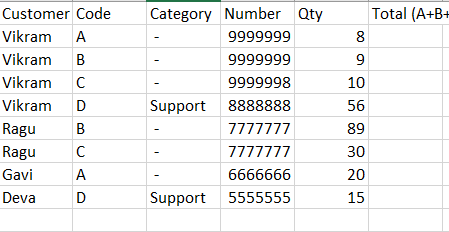In Column(Customer name and Number) if it’s the Same I add Column (code) (A+B+C)only, not add (Code D) that answer is stored in Var(Total).

This Var data How to append the data from the Data table to Excel.
Before writing the Excel I have to append the data in the Existing Excel sheet (Data table to append)

Please check and help me with this topic

Thanks
Vikram

Hi @vr10 ,

Could you provide an Expected Output data for the Input Provided either in the form of a Screenshot or as an Excel file, We will be able to help you better in this way suggesting the appropriate solution for your case.

This is the sample file I need to answer in F Column.
I did the calculation part in Studio using some conditions that answer stored in a variable (Total).

Could help me this

Thanks
Vikram

As mentioned the Screenshot provided is the Input, Could you fill the Total Column manually and let us know the values (Final Output required). We would need to understand what is meant by (A+B+C), this is not quite clear.

I have data from Columns A to E,

I required answers like G And H

(I got some answers but If I used to write cell activity to write it took too much time is it possible to do a simple method ) like directly appended to the data table to excel.

Thanks
Vikram.R

I have to add only if it (Desktop, laptop, and Television Qty) not required mobile Qty.

after adding qty I will show in the next column what and all I add (like the Remarks Column shown)

Thanks

Could you Check with the below Steps :

1. Use `Read Range` Workbook Activity to read the data in the Excel sheet as a Datatable, say `DT`. Here, it is assumed that the Data does not contain the columns `Total` and `Remarks` for simplicity calculation purpose.
2. Next, we will Clone the Input datatable to prepare the Output Datatable say, `OutputDT`. We can clone in the below way :
``````OutputDT = DT.Clone
``````

`OutputDT` is of type Datatable.

1. Next use two `Add Data Column` Activities to add the Columns `Total` and `Remarks` to the Output Datatable, `OutputDT`.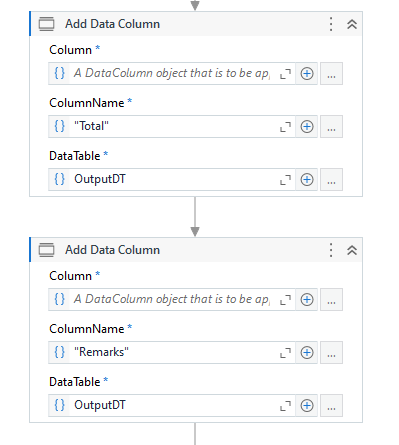2. Next, we will calculate the `Total` and `Remarks` column values based on the Condition mentioned and add the values to the Output datatable using the below Linq Expression in an `Assign` Activity :

``````OutputDT = (From d1 In DT.AsEnumerable
Group d1 By k=d1(0).toString.Trim Into grp=Group
From g In grp.DefaultIfEmpty
Let onlyReqGrp = grp.Where(Function(x){"Laptop","Desktop","Television"}.Any(Function(y)y.ToString.ToLower.Equals(x("Code").ToString.ToLower))).ToArray
Let total = onlyReqGrp.Sum(Function(x)CDbl(x("Qty"))).ToString+"("+String.Join("+",onlyReqGrp.Select(Function(x)x("Qty").ToString).ToArray)+")"
Let remarks = "("+String.Join("+",onlyReqGrp.Select(Function(x)x("Code").ToString))+")"
Select ra = If(Not({"Laptop","Desktop","Television"}.Any(Function(x)g("Code").ToString.ToLower.Equals(x.ToLower))),g.ItemArray.Concat({"-","-"}).ToArray,g.ItemArray.Concat({total,remarks}).ToArray)
``````
1. Then, You could write this `OutputDT` datatable to an Excel sheet using `Write Range` Workbook Activity.

Input :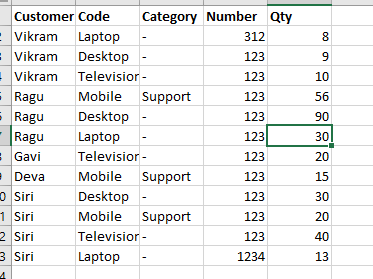Output :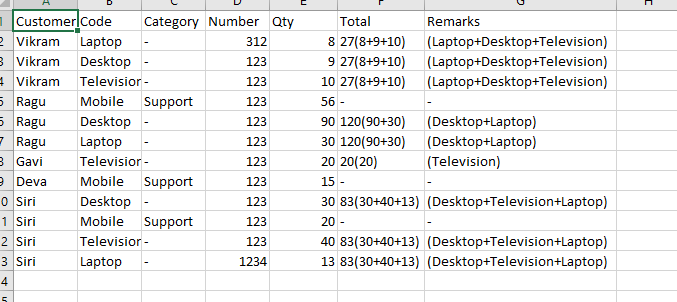Let us know if you are facing any issues in implementing this. Do provide details of it so that we can help further.

1 Like

Thank you so much for helping. i will implement this code if anything i will let you know…

1 Like

Hi,

Thanks a lot Superman.

-previous one

Sorry for Changing. Code is working partially.

Please can you do some changes.
like that i need data

–New one

1. A to E column i have Data
2.instead of customer name we have to consider as number as same.

3.same Number = (Code =only laptop, desktop, Television) i get total qty in G., (In G column just i need total like 17 not required (8+9) if it’s not there these just i have to showed as 0

1. code =Mob and Category =Support i will get qty in F Column. if it’s not there just showed as 0

5.In H Cloumn i need Support /Total (like percentage)

6.In Remarks Column whatever i added in (total column like these 3 (laptop, desktop, Television) and if it’s not there just i have to show as No values.)

Because of these i am working last 1 month but i didn’t get answer but your completely worked in only 1 code ,i used so many conditions. It’s too length your did Amazing.

If it’s possible, could you share Xaml.(its very useful)

Thanks a lot Superman.

Hi @vr10 ,

Could you provide the Input in the form of an Excel sheet and also it’s Expected Output, it would be better to check directly with the code what is happening and what you require, so that we can adapt to the requirement faster.

Also could you confirm if we could keep the `Total`, `Percentage` and `Remarks` columns out from the Input data, as this would be an advantage when creating the output data. However, let us know if it is required to be present in the Input data as well and we cannot skip it.

Also, noticing the `Telivision` is spelt wrongly if you compare the expression that I have provided and the word in Excel sheet.

Maybe correcting that is what is needed ?

1 Like

Hi ,

Input File:

Output File:

I need F, G , H , I Columns (like Support, Total, Percentage and Remarks).

1.based on number (Coloumn) we will work.(Because customer name same but number as changed so)

2.If its number is included (laptop, Desktop and Television) That’s total Qty add and save to Total column. (G column) like same whatever we added we have to show in Remarks Column.

Example: 1st Column number is 12345 (tat’s in 3 Columns same) its included laptop and Desktop only (code Column)
these 2 total Qty (8+9) 17 its should shown in Total Column.
we added qty laptop and Desktop only for these we have to shown in Remarks Column. like (Laptop + Desktop)

if it’s not included any of these 3(laptop + Desktop + Television) just we have to show as “0” for that number like Example 6th row (Number 12346)

1. if its Code(Coloumn) = Mobile and Category (Column) = Support these
2 matches that qty amount we have shown in Support (Column) like 1st one shown in output data.
if its not included support qty that number just we have to shown as “0”. ( Example like 5th row )

2. After getting 2 Qty we will divide (like Support /Total)

previous code is almost its nearest just we have to add some new things that’s it.

Please check and revert back to me

Thanks for Helping.

Hi @vr10 ,

There is a slight confusion with the `Support` Column. Is it supposed to be present in the Input data as well ? As the `Support` Column calculation either is not mentioned or is not clear in your explanation provided above.

Also, I believe for Mobile Support the Total and Percentage values would be 0 ?

This is the Output received :

Also, If you already do have a workflow created, the modifications would be to add another `Add Data Column` Activity with the Column name as `Percentage` after adding the `Total` Column.

Then you could use the below Expression instead of the expression suggested above :

``````(From d1 In DT.AsEnumerable
Group d1 By k=d1("Number").toString.Trim Into grp=Group
From g In grp.DefaultIfEmpty
Let onlyReqGrp = grp.Where(Function(x){"Laptop","Desktop","Telivision"}.Any(Function(y)y.ToString.ToLower.Equals(x("Code").ToString.ToLower))).ToArray
Let total = onlyReqGrp.Sum(Function(x)CDbl(x("Qty")))
Let percentage = Math.Round((CDbl(g("Support").ToString)/total)*100)
Let remarks = "("+String.Join("+",onlyReqGrp.Select(Function(x)x("Code").ToString))+")"
Select ra = If(Not({"Laptop","Desktop","Telivision"}.Any(Function(x)g("Code").ToString.ToLower.Equals(x.ToLower))),g.ItemArray.Concat({"0","0%","No values"}).ToArray,g.ItemArray.Concat({total,percentage.ToString+"%",remarks}).ToArray)
``````

Let us know if you still need a workflow or if you were able to make it work.

1 Like

I am getting This Error:
Column ‘Support’ does not belong to table DataTable.

“Support Column” Nothing Calculation is there Just we need to add (if its Code Coloumn is matches “MOB” and category coloumn is matches support (both are same) these 2 are same that qty should write in Support Coloumn.like example:
4th , 6th row as shown in output file. if its not matches for that number we have to write “0” like 12th number shown in output file.

Sorry for Asking again and again please check and let me know this one concept.

Thanks

Still not able to understand clearly.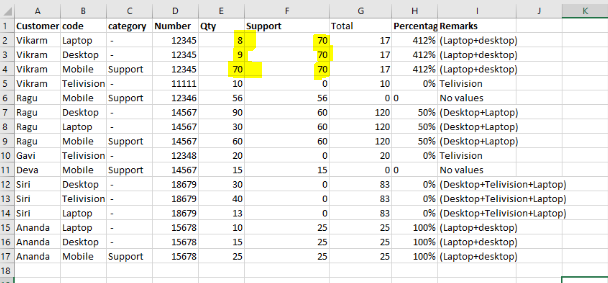According to the Required Output that you have provided, there isn’t a Formula observed for the `Support` column values.

The logic that we could identify is that for `Mobile Support` values we just need to copy the Qty value itself but what about the other Category values ?

How was the value 70 Applied for `Laptop` and `Desktop` categories ?

1 Like

Its Exactly Correct: we could identify is that for `Mobile Support` values we just need to copy the Qty value itself

Example:
Check the 14567 - Number(coloumn) same Number., 3 codes is there(laptop+desktop) added and that qty amount we will showed in total coloumn like (90+30) its 120.

like that For MoBile-support qty its showing as support coloumn hence number is same (14567)for all 3 codes

based on Number we have to shown (where ever number as same just we to shown like Total Coloumn showed(90+30=120) just we have to show 60

If its not there Mob - support is that number just we have to shown 0 like 11111

Eaxmple:

Thanks

I believe I was able to get the output as required. However, do confirm after you have tested with the modifications below :

Change the Expression to the below :

``````(From d1 In DT.AsEnumerable
Group d1 By k=d1("Number").toString.Trim Into grp=Group
From g In grp.DefaultIfEmpty
Let onlyReqGrp = grp.Where(Function(x){"Laptop","Desktop","Telivision"}.Any(Function(y)y.ToString.ToLower.Equals(x("Code").ToString.ToLower))).ToArray
Let mobileSupportGrp = grp.Where(Function(x)Not({"Laptop","Desktop","Telivision"}.Any(Function(y)y.ToString.ToLower.Equals(x("Code").ToString.ToLower)))).ToArray
Let support = If(mobileSupportGrp.Any,mobileSupportGrp.Sum(Function(x)CDbl(x("Qty"))),0)
Let total = onlyReqGrp.Sum(Function(x)CDbl(x("Qty")))
Let percentage = If(total=0 OrElse support=0,0,Math.Round((CDbl(support)/total)*100))
Let remarks = If(onlyReqGrp.Any,"("+String.Join("+",onlyReqGrp.Select(Function(x)x("Code").ToString))+")","No Values")
Select ra = g.ItemArray.Concat({support,total,percentage.ToString+"%",remarks}).ToArray
``````

Also, You would require to use `Add Data Column` Activity and add the column `"Support"` to the `OutputDT` datatable, before adding the column `"Total"`.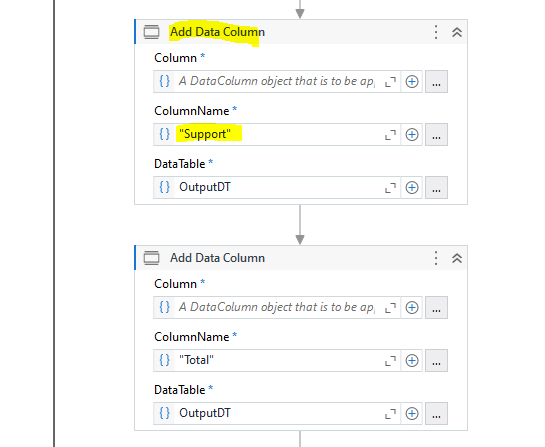Let us know if you were able to get the expected result after these modifications.

1 Like

Thanks for helping step by step Superman Code is working Fine .(I struggled so many ways but not working this much Easy ,your code is Extremely Nice.)

Thanks for UiPath Forum as well.

If any Thing required i take your help. :slight smile:

1 Like

Hi ,

Let mobileSupportGrp = grp.Where(Function(x)Not({“Laptop”,“Desktop”,“Telivision”}.Any(Function(y)y.ToString.ToLower.Equals(x(“Code”).ToString.ToLower)))).ToArray
Let support = If(mobileSupportGrp.Any,mobileSupportGrp.Sum(Function(x)CDbl(x(“Qty”))),0)

Thanks

The `mobileSupportGrp` is like a variable created within that query, we cannot add spaces to that part when declaring it with `Let`. Could you let us know why do you want to add that or change that name ?

ohh okay Thanks.

I have to change some time one more is there like that same so (Then if i want any new i can’t change it ha)

Actually instead of “mobileSupport” place i have add these both i have to change as per requirement so “ServicesPremier Support” and “ServiceDeployment/Recovery Services”.

I send sample file so other one its confidential so , i am able to change like (“Laptop"DesktoP"Telivision”) aprt from “Mobservice” everythinng i can get Answer so.

is it any alternative to change as per requirement…? please check and let me know is it possible.

Thanks